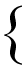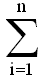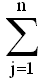# Formulate Assignment Problem

The assignment problem is a special type of transportation problem, where the objective is to minimize the cost or time of completing a number of jobs by a number of persons.

In other words, when the problem involves the allocation of n different facilities to n different tasks, it is often termed as an assignment problem.

The model's primary usefulness is for planning. The assignment problem also encompasses an important sub-class of so-called shortest- (or longest-) route models. The assignment model is useful in solving problems such as, assignment of machines to jobs, assignment of salesmen to sales territories, travelling salesman problem, etc.

It may be noted that with n facilities and n jobs, there are n! possible assignments. One way of finding an optimal assignment is to write all the n! possible arrangements, evaluate their total cost, and select the assignment with minimum cost. But, due to heavy computational burden this method is not suitable. This chapter concentrates on an efficient method for solving assignment problems that was developed by a Hungarian mathematician D.Konig.

"A mathematician is a device for turning coffee into theorems." -Paul Erdos

## Formulation of an assignment problem

Suppose a company has n persons of different capacities available for performing each different job in the concern, and there are the same number of jobs of different types. One person can be given one and only one job. The objective of this assignment problem is to assign n persons to n jobs, so as to minimize the total assignment cost. The cost matrix for this problem is given below:The structure of an assignment problem is identical to that of a transportation problem.

To formulate the assignment problem in mathematical programming terms, we define the activity variables as

 xij =1 if job j is performed by worker i 0 otherwise

for i = 1, 2, ..., n and j = 1, 2, ..., n

In the above table, cij is the cost of performing jth job by ith worker.

## Generalized Form of an Assignment Problem

The optimization model is

Minimize c11x11 + c12x12 + ------- + cnnxnn

subject to
xi1 + xi2 +..........+ xin = 1          i = 1, 2,......., n
x1j + x2j +..........+ xnj = 1          j = 1, 2,......., n

xij = 0 or 1

#### In Σ Sigma notation

minimizecijxij

subject toxij = 1 for i = 1, 2, ....., nxij = 1 for j = 1, 2, ....., n

xij = 0 or 1 for all i and j

An assignment problem can be solved by transportation methods, but due to high degree of degeneracy the usual computational techniques of a transportation problem become very inefficient. Therefore, a special method is available for solving such type of problems in a more efficient way.

### Assumptions in Assignment Problem

• Number of jobs is equal to the number of machines or persons.
• Each man or machine is assigned only one job.
• Each man or machine is independently capable of handling any job to be done.
• Assigning criteria is clearly specified (minimizing cost or maximizing profit).

The assignment problem is one of the fundamental combinatorial optimization problems in the branch of optimization or operations research in mathematics. It consists of finding a maximum weight matching (or minimum weight perfect matching) in a weightedbipartite graph.

In its most general form, the problem is as follows:

The problem instance has a number of agents and a number of tasks. Any agent can be assigned to perform any task, incurring some cost that may vary depending on the agent-task assignment. It is required to perform all tasks by assigning exactly one agent to each task and exactly one task to each agent in such a way that the total cost of the assignment is minimized.

If the numbers of agents and tasks are equal and the total cost of the assignment for all tasks is equal to the sum of the costs for each agent (or the sum of the costs for each task, which is the same thing in this case), then the problem is called the linear assignment problem. Commonly, when speaking of the assignment problem without any additional qualification, then the linear assignment problem is meant.

## Algorithms and generalizations

The Hungarian algorithm is one of many algorithms that have been devised that solve the linear assignment problem within time bounded by a polynomial expression of the number of agents. Other algorithms include adaptations of the primal simplex algorithm, and the auction algorithm.

The assignment problem is a special case of the transportation problem, which is a special case of the minimum cost flow problem, which in turn is a special case of a linear program. While it is possible to solve any of these problems using the simplex algorithm, each specialization has more efficient algorithms designed to take advantage of its special structure.

When a number of agents and tasks is very large, a parallel algorithm with randomization can be applied. The problem of finding minimum weight maximum matching can be converted to finding a minimum weight perfect matching. A bipartite graph can be extended to a complete bipartite graph by adding artificial edges with large weights. These weights should exceed the weights of all existing matchings to prevent appearance of artificial edges in the possible solution. As shown by Mulmuley, Vazirani & Vazirani (1987), the problem of minimum weight perfect matching is converted to finding minors in the adjacency matrix of a graph. Using the isolation lemma, a minimum weight perfect matching in a graph can be found with probability at least ½. For a graph with n vertices, it requirestime.

## Example

Suppose that a taxi firm has three taxis (the agents) available, and three customers (the tasks) wishing to be picked up as soon as possible. The firm prides itself on speedy pickups, so for each taxi the "cost" of picking up a particular customer will depend on the time taken for the taxi to reach the pickup point. The solution to the assignment problem will be whichever combination of taxis and customers results in the least total cost.

However, the assignment problem can be made rather more flexible than it first appears. In the above example, suppose that there are four taxis available, but still only three customers. Then a fourth dummy task can be invented, perhaps called "sitting still doing nothing", with a cost of 0 for the taxi assigned to it. The assignment problem can then be solved in the usual way and still give the best solution to the problem.

Similar adjustments can be done in order to allow more tasks than agents, tasks to which multiple agents must be assigned (for instance, a group of more customers than will fit in one taxi), or maximizing profit rather than minimizing cost.

## Formal mathematical definition

The formal definition of the assignment problem (or linear assignment problem) is

Given two sets, A and T, of equal size, together with a weight functionC : A × TR. Find a bijectionf : AT such that the cost function:is minimized.

Usually the weight function is viewed as a square real-valued matrixC, so that the cost function is written down as:The problem is "linear" because the cost function to be optimized as well as all the constraints contain only linear terms.

The problem can be expressed as a standard linear program with the objective functionsubject to the constraintsThe variablerepresents the assignment of agentto task, taking value 1 if the assignment is done and 0 otherwise. This formulation allows also fractional variable values, but there is always an optimal solution where the variables take integer values. This is because the constraint matrix is totally unimodular. The first constraint requires that every agent is assigned to exactly one task, and the second constraint requires that every task is assigned exactly one agent.